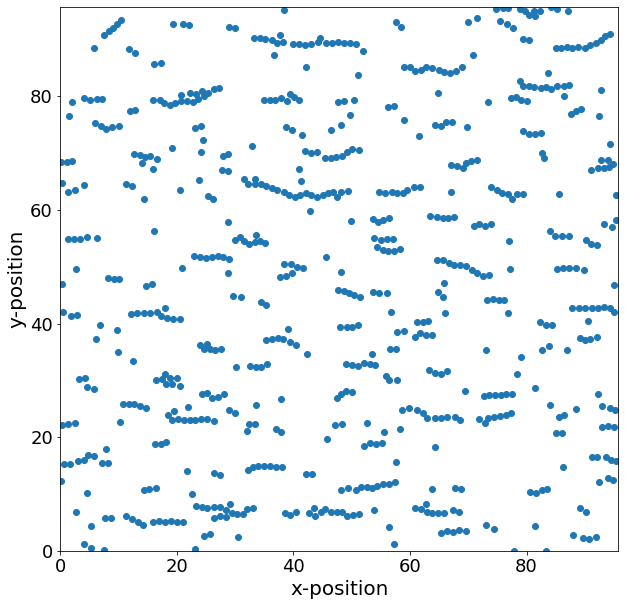# Ferrofluid - Part 2¶

Remark: The equilibration and sampling times used in this tutorial would be not sufficient for scientific purposes, but they are long enough to get at least a qualitative insight of the behaviour of ferrofluids. They have been shortened so we achieve reasonable computation times for the purpose of a tutorial.

## Applying an external magnetic field¶

In this part we want to investigate the influence of a homogeneous external magnetic field exposed to a ferrofluid system.

We import all necessary packages and check for the required ESPResSo features

In :
import espressomd
import espressomd.magnetostatics

espressomd.assert_features(['DIPOLES', 'DP3M', 'LENNARD_JONES'])

%matplotlib inline
import matplotlib.pyplot as plt

In :
plt.rcParams.update({'font.size': 18})
import numpy as np
import tqdm


and set up the simulation parameters where we introduce a new dimensionless parameter

\begin{equation} \alpha = \frac{\mu B}{k_{\text{B}}T} = \frac{\mu \mu_0 H}{k_{\text{B}}T} \end{equation}

which is called Langevin parameter. We intentionally choose a relatively high volume fraction $\phi$ and dipolar interaction parameter $\lambda$ to clearly see the influence of the dipole-dipole interaction

In :
# Lennard-Jones parameters
LJ_SIGMA = 1.
LJ_EPSILON = 1.
LJ_CUT = 2**(1. / 6.) * LJ_SIGMA

# Particles
N_PART = 700

# Area fraction of the mono-layer
PHI = 0.06

# Dipolar interaction parameter lambda = MU_0 m^2 /(4 pi sigma^3 kT)
DIP_LAMBDA = 4.

# Temperature
KT = 1.0

# Friction coefficient
GAMMA = 1.0

# Time step
TIME_STEP = 0.01

# Langevin parameter ALPHA = MU_0 m H / kT
ALPHA = 10.

# vacuum permeability
MU_0 = 1.


Now we set up the system. As in part I, the orientation of the dipole moments is set directly on the particles, whereas the magnitude of the moments is taken into account when determining the prefactor of the dipolar P3M (for more details see part I).

Hint: It should be noted that we seed both the Langevin thermostat and the random number generator of numpy. The latter means that the initial configuration of our system is the same every time this script will be executed. As the time evolution of the system depends not solely on the Langevin thermostat but also on the numeric accuracy and DP3M as well as DLC (the tuned parameters are slightly different every time) it is only partly predefined. You can change the seeds to simulate with a different initial configuration and a guaranteed different time evolution.

In :
# System setup
box_size = (N_PART * np.pi * (LJ_SIGMA / 2.)**2. / PHI)**0.5

print("Box size", box_size)
# Note that the dipolar P3M and dipolar layer correction need a cubic
# simulation box for technical reasons.
system = espressomd.System(box_l=(box_size, box_size, box_size))
system.time_step = TIME_STEP

# Lennard-Jones interaction
system.non_bonded_inter[0, 0].lennard_jones.set_params(epsilon=LJ_EPSILON, sigma=LJ_SIGMA, cutoff=LJ_CUT, shift="auto")

# Random dipole moments
np.random.seed(seed=1)
dip_phi = 2. * np.pi * np.random.random((N_PART, 1))
dip_cos_theta = 2 * np.random.random((N_PART, 1)) - 1
dip_sin_theta = np.sin(np.arccos(dip_cos_theta))
dip = np.hstack((
dip_sin_theta * np.sin(dip_phi),
dip_sin_theta * np.cos(dip_phi),
dip_cos_theta))

# Random positions in the monolayer
pos = box_size * np.hstack((np.random.random((N_PART, 2)), np.zeros((N_PART, 1))))

particles = system.part.add(pos=pos, rotation=N_PART * [(True, True, True)], dip=dip, fix=N_PART * [(False, False, True)])

# Remove overlap between particles by means of the steepest descent method
system.integrator.set_steepest_descent(
f_max=0, gamma=0.1, max_displacement=0.05)

while system.analysis.energy()["total"] > 5 * KT * N_PART:
system.integrator.run(20)

# Switch to velocity Verlet integrator
system.integrator.set_vv()
system.thermostat.set_langevin(kT=KT, gamma=GAMMA, seed=1)

# tune verlet list skin
system.cell_system.tune_skin(min_skin=0.4, max_skin=2., tol=0.2, int_steps=100)

# Setup dipolar P3M and dipolar layer correction (DLC)
dp3m = espressomd.magnetostatics.DipolarP3M(accuracy=5E-4, prefactor=DIP_LAMBDA * LJ_SIGMA**3 * KT)
mdlc = espressomd.magnetostatics.DLC(actor=dp3m, maxPWerror=1E-4, gap_size=box_size - LJ_SIGMA)

# tune verlet list skin again
system.cell_system.tune_skin(min_skin=0.4, max_skin=2., tol=0.2, int_steps=100)

# print skin value
print(f'tuned skin = {system.cell_system.skin:.2f}')

Box size 95.72344839677595
tuned skin = 0.55


We now apply the external magnetic field which is

\begin{equation} B = \mu_0 H = \frac{\alpha~k_{\text{B}}T}{\mu} \end{equation}

As only the current orientation of the dipole moments, i.e. the unit vector of the dipole moments, is saved in the particle list but not their magnitude, we have to use $B\cdot \mu$ as the strength of the external magnetic field. We will apply the field in x-direction using the class constraints of ESPResSo

In :
# magnetic field times dipole moment
H_dipm = ALPHA * KT
H_field = [H_dipm, 0, 0]


## Exercise:¶

Define a homogenous magnetic field constraint using H_field and add it to system's contraints.

In :
H_constraint = espressomd.constraints.HomogeneousMagneticField(H=H_field)

Out:
<espressomd.constraints.HomogeneousMagneticField at 0x7f117d178e50>

Equilibrate the system.

In :
# Equilibrate
print("Equilibration...")
equil_rounds = 10
equil_steps = 100
for i in tqdm.trange(equil_rounds):
system.integrator.run(equil_steps)

Equilibration...

100%|██████████| 10/10 [00:01<00:00,  5.34it/s]


Now we can visualize the current state and see that the particles mostly create chains oriented in the direction of the external magnetic field. Also some monomers should be present.

In :
plt.figure(figsize=(10, 10))
plt.xlim(0, box_size)
plt.ylim(0, box_size)
plt.xlabel('x-position', fontsize=20)
plt.ylabel('y-position', fontsize=20)
plt.plot(particles.pos_folded[:, 0], particles.pos_folded[:, 1], 'o')
plt.show()## Video of the development of the system¶

You may want to get an insight of how the system develops in time. Thus we now create a function which will save a video and embed it in an html string to create a video of the systems development

In :
import matplotlib.pyplot as plt

In :
import matplotlib.animation as animation

In [ ]:
import tempfile
import base64

VIDEO_TAG = """<video controls>
<source src="data:video/x-m4v;base64,{0}" type="video/mp4">
Your browser does not support the video tag.
</video>"""

def anim_to_html(anim):
if not hasattr(anim, '_encoded_video'):
with tempfile.NamedTemporaryFile(suffix='.mp4') as f:
anim.save(f.name, fps=20, extra_args=['-vcodec', 'libx264'])
with open(f.name, "rb") as g:
anim._encoded_video = base64.b64encode(video).decode('ascii')
plt.close(anim._fig)
return VIDEO_TAG.format(anim._encoded_video)

animation.Animation._repr_html_ = anim_to_html

def init():
# Set x and y range
ax.set_ylim(0, box_size)
ax.set_xlim(0, box_size)
xdata, ydata = [], []
part.set_data(xdata, ydata)
return part,

def run(i):
system.integrator.run(50)

# Save current system state as a plot
xdata, ydata = particles.pos_folded[:, 0], particles.pos_folded[:, 1]
ax.figure.canvas.draw()
part.set_data(xdata, ydata)
print(f'progress: {(i + 1):3.0f}%', end='\r')
return part,


We now can start the sampling over the animation class of matplotlib

In :
fig, ax = plt.subplots(figsize=(10, 10))
part, = ax.plot([], [], 'o')

animation.FuncAnimation(fig, run, frames=100, blit=True, interval=0, repeat=False, init_func=init)

progress: 100%

Out: